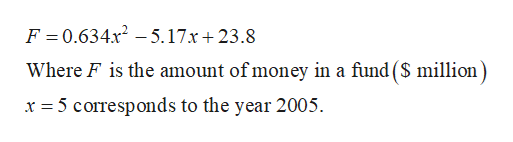# The amount of money in a fund F (in millions of dollars) can be approximated by the equation F=.634x2-5.17x+23.8, where x=5 corresponds to the year 2005. What year produced \$72 million?

Question
1 views

The amount of money in a fund F (in millions of dollars) can be approximated by the equation F=.634x2-5.17x+23.8, where x=5 corresponds to the year 2005. What year produced \$72 million?

check_circle

Step 1

Consider the given...help_outlineImage TranscriptioncloseF = 0.634x2 -5.17x+ 23.8 Where F is the amount of money in a fund (S million) x =5 corresponds to the year 2005. fullscreen

### Want to see the full answer?

See Solution

#### Want to see this answer and more?

Solutions are written by subject experts who are available 24/7. Questions are typically answered within 1 hour.*

See Solution
*Response times may vary by subject and question.
Tagged in

### Other